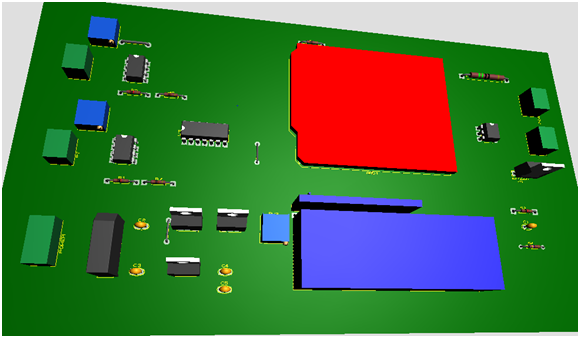# Flexible Ac Transmitter System Using TSR

0
1349The aim of the project is to achieve FACTS (Flexible AC transmission system) using TSR (Thyristor Switch Reactance). This is done when the receiving end voltage is low while charging the transmission line. In the presence of a small load, a small current flows through the transmission line there for shut capacitance becomes more effective. Due to the Ferranti Effect, the voltage at receiving end increases two times than the voltage at sending end.

Every electrical apparatus can handle certain voltage beyond that particular voltage level it will burn out. The increase in receiving end voltage due to the Ferranti effect can damage the electrical equipment. So, to control it we used a shunt reactor at the receiving end.

## Working and Explanation

FACTS using TSR requires an operational amplifier that will generate lead time between zero voltage pulse and zero current pulse. This lead time is given to two interrupt pins of Arduino Uno. The Arduino program then initiates the shunt reactors for compensating for the voltage. The SCRs are arranged in series with Arduino Uno through optical isolation and are used for switching reactor.

### Components used:

The following components are used in Arduino based Ferranti effect over come using thyristor

• Thyristor
• Thyristor Switched Reactor
• Potential Transformer
• Current Transformer
• ZMCT103C AC current Sensor
• Capacitor
• Voltage regulator
• LCD
• Arduino Uno
• Zero-Crossing Detector
• Cables and Connectors
• Lamp
• Switch

## Circuit and Results (Flexible Ac Transmitter System Using TSR)

The image below is simulation of Facts using TSR

## Coding (Flexible Ac Transmitter System Using TSR)

``````// include the library code:
#include <LiquidCrystal.h>
// initialize the library by associating any needed LCD interface pin
// with the arduino pin number it is connected to
const int rs = 8, en = 9, d4 = 10, d5 = 11, d6 = 12, d7 = 13;
LiquidCrystallcd(rs, en, d4, d5, d6, d7);
int triac = A5;
int button = A1;
void setup()
{
// set up the LCD's number of columns and rows:
lcd.begin(16, 2);
// Print a message to the LCD.
pinMode(button,INPUT);
pinMode(triac,OUTPUT);
digitalWrite(triac,LOW);
}

void loop()
{
// set the cursor to column 0, line 1
// (note: line 1 is the second row, since counting begins with 0):
lcd.setCursor(0, 1);
lcd.print("PF:");
int duration = pulseIn(lead,HIGH); // millisecs
lcd.setCursor(10, 1);
lcd.print(duration);
float duration1=(duration/1000);
float si=(duration1/20); // when freq = 50 hz , ten T =20MS
float phi=(si*360);
float power_factor=abs(cos(phi/57.2));

if(power_factor> 0.99)
{
lcd.setCursor(0, 0);
lcd.print("turn on power");
delay(2000);
lcd.clear();
}
else
{
// print the number of seconds since reset:
lcd.setCursor(4, 1);
lcd.print(power_factor);

{
lcd.setCursor(0, 0);
lcd.print("   Normal Mode");
digitalWrite(triac,LOW);
delay(2000);
}
else
{
lcd.setCursor(0, 0);
lcd.print("Compensation Mode");
digitalWrite(triac,HIGH);
delay(2000);
}
}}
``````